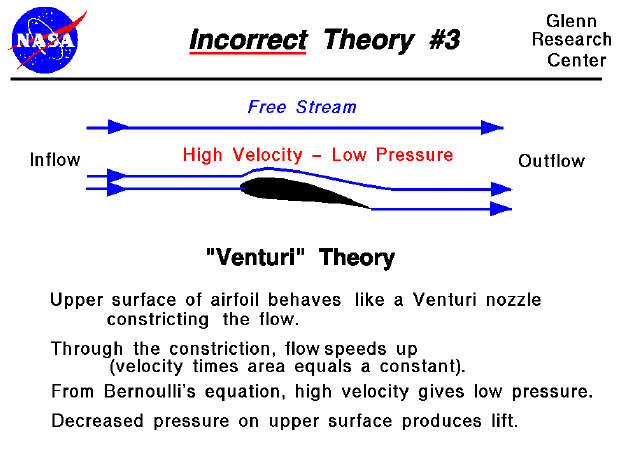There are many theories of how lift is generated. Unfortunately, many of the theories found in encyclopedias, on web sites, and even in some textbooks are incorrect, causing unnecessary confusion for students.

The theory described on this slide is often seen on web sites and in popular literature. The theory is based on a model that the airfoil upper surface is shaped to act as a nozzle which accelerates the flow. This nozzle configuration is known as a Venturi nozzle and it can be analyzed classically. By considering the conservation of mass, the mass flowing past any point on the airfoil (nozzle) is a constant. This leads to the relation that the mass flow rate (the density times the velocity times the area) of the Venturi is a constant. For a constant density, at any point where the area is decreased, the velocity will be increased. Therefore, over the top of the airfoil the velocity will be increased due to the flow constriction. Now, coupling this with Bernoulli's equation, at any point where the area is decreased the pressure will be decreased. The low pressure over the upper surface of the airfoil then produces the lift.

Before considering what is wrong with this theory, let's investigate the actual flow around an airfoil by doing a couple of experiments using a Java simulator which is solving the correct flow equations.

This interactive Java applet shows flow going past a symmetric airfoil. The flow is shown by a series of moving particles. You can change the angle of attack of the airfoil by using a slider, and the angle of attack generates the lift through flow turning. There is also a translating probe with a gage on the simulator which lets you investigate the flow.

This is a secondary Java applet which uses a text box to describe some experiments for the student to perform using the previous applet.

Let's use the information we've just learned to evaluate the various parts of the "Venturi" Theory.

• This theory is based on an analysis of a Venturi nozzle. But an airfoil is not a Venturi nozzle. There is no phantom surface to produce the other half of the nozzle. In our experiments we've noted that the velocity gradually decreases as you move away from the airfoil eventually approaching the free stream velocity. This is not the velocity found along the centerline of a nozzle which is typically higher than the velocity along the wall.
• The Venturi analysis cannot predict the lift generated by a flat plate. The leading edge of a flat plate presents no constriction to the flow so there is really no "nozzle" formed. One could argue that a "nozzle" occurs when the angle of the flat plate is negative. But as we have seen in Experiment #2, this produces a negative lift. The velocity actually slows down on the upper surface at a negative angle of attack; it does not speed up as expected from the nozzle model.
• This theory deals with only the pressure and velocity along the upper surface of the airfoil. It neglects the shape of the lower surface. If this theory were correct, we could have any shape we want for the lower surface, and the lift would be the same. This obviously is not the way it works - the lower surface does contribute to the lift generated by an airfoil. (In fact, one of the other incorrect theories proposed that only the lower surface produces lift!)
• The part of the theory about Bernoulli's equation and a difference in pressure existing across the airfoil is correct. In fact, this theory is very appealing because there are parts of the theory that are correct. In our discussions on pressure-area integration to determine the force on a body immersed in a fluid, we mentioned that if we knew the velocity, we could obtain the pressure and determine the force. The problem with the "Venturi" theory is that it attempts to provide us with the velocity based on an incorrect assumption (the constriction of the flow produces the velocity field). We can calculate a velocity based on this assumption, and use Bernoulli's equation to compute the pressure, and perform the pressure-area calculation and the answer we get does not agree with the lift that we measure for a given airfoil.Guided Tours

Back to top

Go to...

Beginner's Guide Home Page

byTom Benson
Please send suggestions/corrections to: benson@grc.nasa.gov